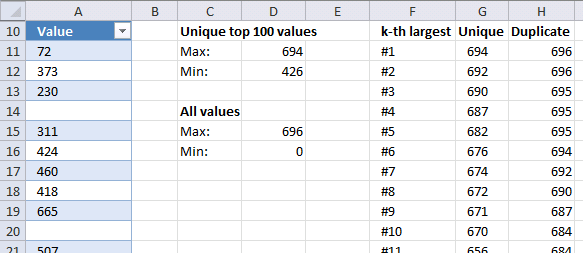Author: Oscar Cronquist Article last updated on October 15, 2018

How to find Min and Max numeric values in a range of cells that have duplicate numbers and blanks, but only want to find the Min and Max on the largest/top 100 non-duplicate values.Array formula in cell D11:

=MAX(IF((COUNTIF(Table1[Value],Table1[Value])<>1)+(Table1[Value]=""),"",Table1[Value]))

Array formula in cell D12:

=LARGE(IF((COUNTIF(Table1[Value],Table1[Value])<>1)+(Table1[Value]=""),"",Table1[Value]),100)

Formula in cell D15:

=MAX(Table1[Value])

Formula in cell D16:

=MIN(Table1[Value])

Array formula in cell G11:

=LARGE(IF((COUNTIF(Table1[Value],Table1[Value])<>1)+(Table1[Value]=""),"",Table1[Value]),ROW(A1))

### Explaining array formula in cell D11

Step 1 - Count the number of cells within a range that meet a given condition

COUNTIF(Table1[Value],Table1[Value])

returns

{1;1;1;0;1;1;1;1;1;...}

Step 2 - Check if value in array is a duplicate

COUNTIF(Table1[Value],Table1[Value])<>1

becomes

{1;1;1;0;1;1;1;1;1;...}<>1

and returns

{FALSE;FALSE;FALSE;TRUE;FALSE;FALSE;FALSE;FALSE;FALSE;...}

Step 3 - Check if table value is a blank

(Table1[Value]="")

returns

{FALSE;FALSE;FALSE;TRUE;FALSE;FALSE;FALSE;FALSE;FALSE; ...}

Step 4 - Return table value if conditions are met

IF((COUNTIF(Table1[Value],Table1[Value])<>1)+(Table1[Value]=""),"",Table1[Value])

becomes

IF({FALSE;FALSE;FALSE;TRUE;FALSE;FALSE;FALSE;FALSE;FALSE; ...}, "", {72;373;230;0;311;424;460;418;665})

and returns

{72;373;230;"";311;424;460;418;665;...}

Step 5 - Return max value

MAX(IF((COUNTIF(Table1[Value],Table1[Value])<>1)+(Table1[Value]=""),"",Table1[Value]))

becomes

=MAX({72;373;230;"";311;424;460;418;665;...})

and returns 694 in cell D11.